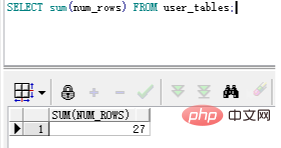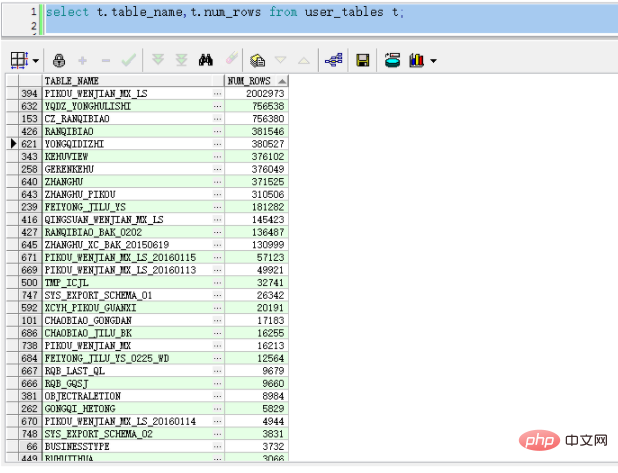﻿ oracle怎样查询数据条数 - 自由资讯

# oracle怎样查询数据条数## oracle怎样查询数据条数

`SELECT sum(num_rows) FROM user_tables;````declare
v_tName varchar(50);
v_sqlanalyze varchar(500);
v_num number;
v_sql varchar(500);
cursor c1
is
select table_name from user_tables;
begin
open c1;
loop
fetch c1 into v_tName;
if c1%found then

v_sqlanalyze :='analyze table  '||v_tName||'  estimate statistics';
execute immediate v_sqlanalyze;
v_sql := 'select NUM_ROWS  from user_tables where table_name =upper('''||v_tName||''')';

execute immediate v_sql into  v_num;
dbms_output.put_line('表名： '||v_tName||' 行数： '||v_num);
else
exit;
end if;
end loop;
end;```

﻿